# NC57【施工单物料用料量报表】操作说明

• 功能位置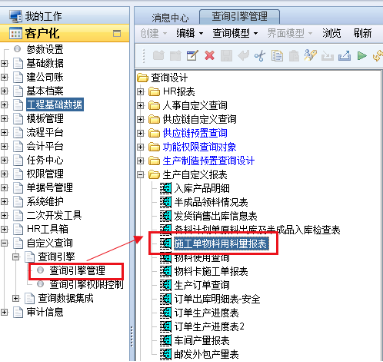• 操作方法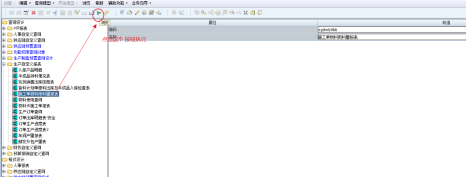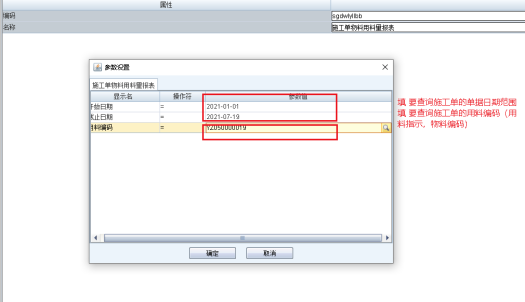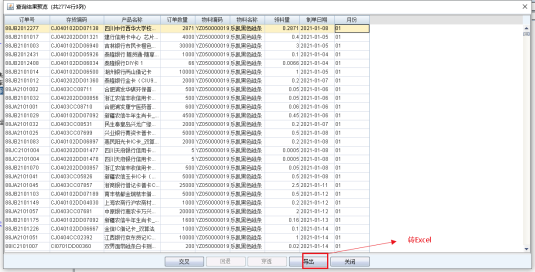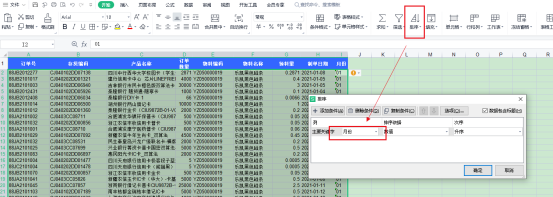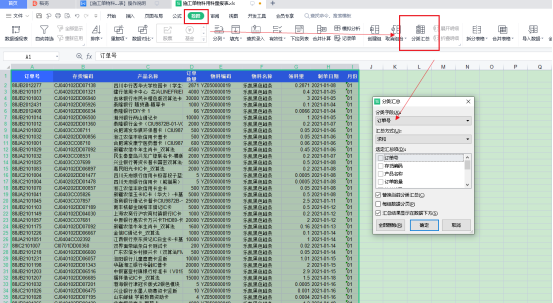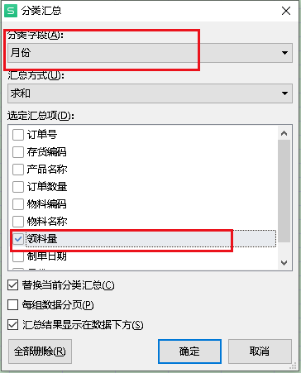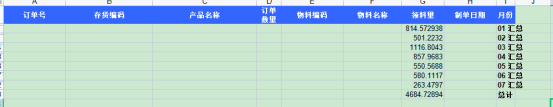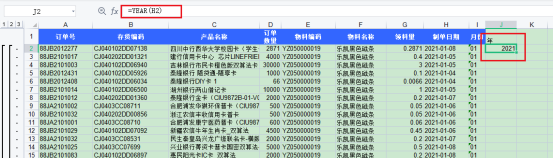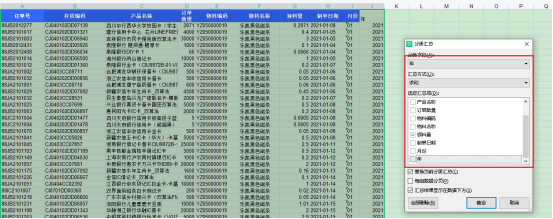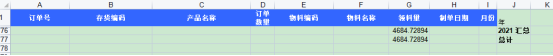• 查询数据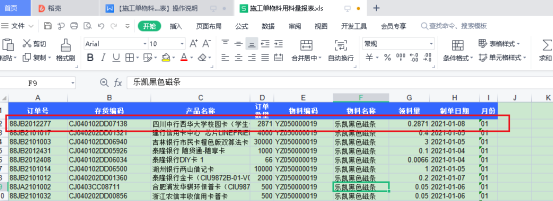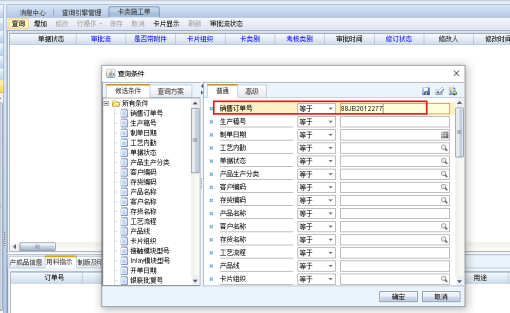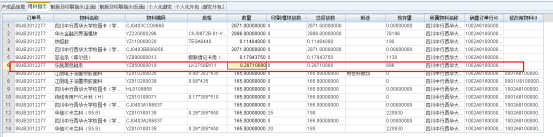• 自定义脚本

select c.ddbillcode as 订单号,c.vdef10 as 生产稿号,c.vdef13 as 产品名称,c.vdef5 as 存货编码,c.vdef4 as 存货名称,c.nnum as 订单数量,
a.procode as 物料编码, a.proname as 物料名称,a.wlnum as 领料量,to_char(to_date(b.dmakedate,'yyyy-mm-dd hh24:mi:ss'),'yyyy-mm-dd') as 制单日期,
to_char(to_date(b.dmakedate,'yyyy-mm-dd hh24:mi:ss'),'mm' ) as 月份

from bf_ksgd_b1 a
left join bf_ksgd_h b on a.pk_ksgd_h=b.pk_ksgd_h
inner join bf_ksgd_b4 c on a.pk_ksgd_h=c.pk_ksgd_h and a.vdef8=c.corder_bid
where nvl(a.dr,0)=0 and nvl(b.dr,0)=0 and nvl(c.dr,0)=0 and b.pk_corp='1002'
and (b.dmakedate>=#startDate# and b.dmakedate<#endDate# )   and a.procode like concat(concat('%',#invcode#),'%')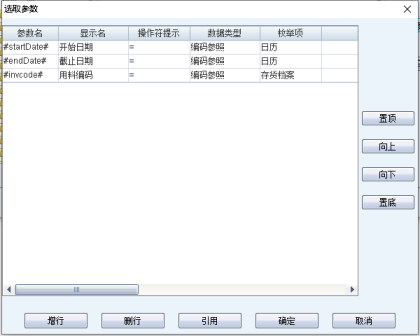THE END